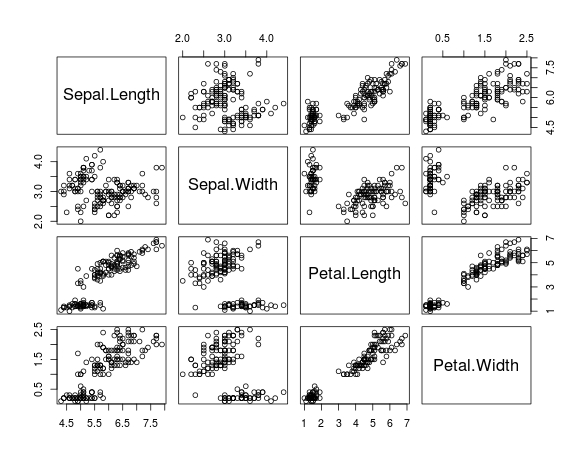# Plotting Correlations in R

A correlation indicates the strength of the relationship between two or more variables.  Plotting correlations allows you tosee if there is a potential relationship between two variables. In this post, we will look at how to plot correlations with multiple variables.

In R, there is a built-in dataset called ‘iris’. This dataset includes information about different types of flowers. Specifically, the ‘iris’ dataset contains the following variables

• Sepal.Length
• Sepal.Width
• Petal.Length
• Petal.Width
• Species

You can confirm this by inputting the following script

```> names(iris)
 "Sepal.Length" "Sepal.Width"  "Petal.Length" "Petal.Width"  "Species"```

We now want to examine the relationship that each of these variables has with each other. In other words, we want to see the relationship of

• Sepal.Length and Sepal.Width
• Sepal.Length and Petal.Length
• Sepal.Length and Petal.Width
• Sepal.Width and Petal.Length
• Sepal.Width and Petal.Width
• Petal.Length and Petal.Width

The ‘Species’ variable will not be a part of our analysis since it is a categorical variable and not a continuous one. The type of correlation we are analyzing is for continuous variables.

We are now going to plot all of these variables above at the same time by using the ‘plot’ function. We also need to tell R not to include the “Species” variable. This is done by adding a subset code to the script. Below is the code to complete this task.

`> plot(iris[-5])`

Here is what we did

1. We use the ‘plot’ function and told R to use the “iris” dataset
2. In brackets, we told R to remove ( – ) the 5th variable, which was species
3. After pressing enter you should have seen the followingThe variable names are placed diagonally from left to right. The x-axis of a plot is determined by variable name in that column. For example,

• The variable of the x-axis of the first column is ‘Sepal.Length”
• The variable of the x-axis of the second column is ‘Sepal.Width”
• The variable of the x-axis of the third column is ‘Petal.Length”
• The variable of the x-axis of the fourth column is ‘Petal.Width”

The y-axis is determined by the variable that is in the same row as the plot. For example,

• The variable of the y-axis of the first column is ‘Sepal.Length”
• The variable of the y-axis of the second column is ‘Sepal.Width”
• The variable of the y-axis of the third column is ‘Petal.Length”
• The variable of the y-axis of the fourth column is ‘Petal.Width”

AS you can see, this is the same information. We will now look at a few examples of plots

• The plot in the first column second row plots “Sepal.Length” as the x-axis and “Sepal.Width” as the y-axis
• The plot in the first column third row plots “Sepal.Length” as the x-axis and “Petal.Length” as the y-axis
• The plot in the first column fourth row plots “Sepal.Length” as the x-axis and “Petal.Width” as the y-axis

Hopefully, you can see the pattern. The plots above the diagonal are mirrors of the ones below. If you are familiar with correlational matrices this should not be surprising.

After a visual inspection, you can calculate the actual statistical value of the correlations. To do so use the script below and you will see the table below after it.

```> cor(iris[-5])
Sepal.Length Sepal.Width Petal.Length Petal.Width
Sepal.Length    1.0000000  -0.1175698    0.8717538   0.8179411
Sepal.Width    -0.1175698   1.0000000   -0.4284401  -0.3661259
Petal.Length    0.8717538  -0.4284401    1.0000000   0.9628654
Petal.Width     0.8179411  -0.3661259    0.9628654   1.0000000```

As you can see, there are many strong relationships between the variables. For example “Petal.Width” and “Petal.Length” has a correlation of .96, which is almost perfect. This means that when “Petal.Width” grows by one unit “Petal.Length” grows by .96 units.

Conclusion

Plots help you to see the relationship between two variables. After visual inspection, it is beneficial to calculate the actual correlation.